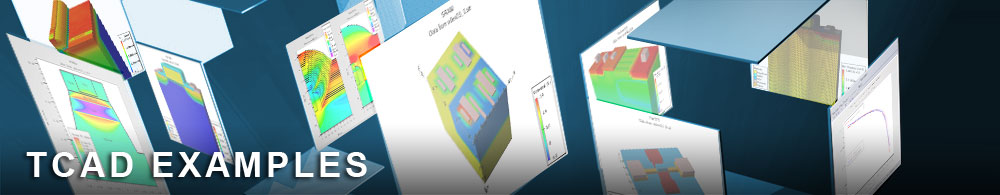Energy Balance and Non-Isothermal Energy Balance

## hbtex04.in : Energy Balance and Non-Isothermal Energy Balance

Requires: Blaze/Giga
Minimum Versions: Atlas 5.28.1.R

This example compares the results of Ic/Vce simulation of an AlGaAs/GaAs HBT using the Energy Balance (EB) and Nonisothermal Energy Balance (NEB) Models. It shows:

• Graded heterojunction AlGaAs/GaAs HBT formation using Atlas syntax
• Specification of energy balance models
• Solution for Ic/Vce characteristics
• Comparative simulation including lattice heat flow

Deep GaAs submicron devices should be simulated using the energy balance Model due to strong velocity overshoot, which may substantially influence device characteristics. For high current levels, the thermal self-heating effects may also play an important role due to the relatively small heat conductivity in GaAs, thereby decreasing mobility and leading to a negative output conductance. This example demonstrates a comparison of Ic-Vce curves obtained with Energy Balance and Nonisothermal Energy Balance Models.

Two Atlas runs are included in this example. The initial stages of each uses Atlas syntax to specify a graded heterojunction HBT. The syntax used is similar to the AlGaAs/GaAs HBT examples described before. The first run proceeds to select and solve self-consistently the classical equations: Poisson's equation and the two carrier continuity equations, and the energy balance equation for electrons. The second run adds the lattice heat flow equation to these four and solves self-consistently using a coupled solver.

The energy balance model is selected by models hcte.el . The most important parameter in energy balance simulation are the relaxation times. These are set on the material statement.

In each run the base bias is ramped to 1.2V. Then contact num=2 current is used to set current boundary conditions on the base electrode. The base current is forced to a value of 15uA/um. After this the collector voltage is ramped to 2.0V to obtain the Ic/Vce curve.

The same set of models is used, except that the solution of the lattice energy balance equation is activated using the syntax models lat.temp . For the AlGaAs region the temperature dependence of the low field mobilities is set by the tmup and tmun parameters on the material statement.

As with all non-isothermal simulation, thermal boundary conditions must be included. These are defined in the thermcontact statement. A value of the thermal resistance is specified at the thermal contact located along the substrate, and thermal isolation conditions are assumed on the all other surfaces.

The results of the two runs can be overlaid in TonyPlot. The simulation with lattice heating shows less current for a given voltage than the isothermal case. The elevated temperature causes mobility reduction in the semiconductor and hence lower currents.

To load and run this example, select the Load button in DeckBuild > Examples. This will copy the input file and any support files to your current working directory. Select the Run button in DeckBuild to execute the example.